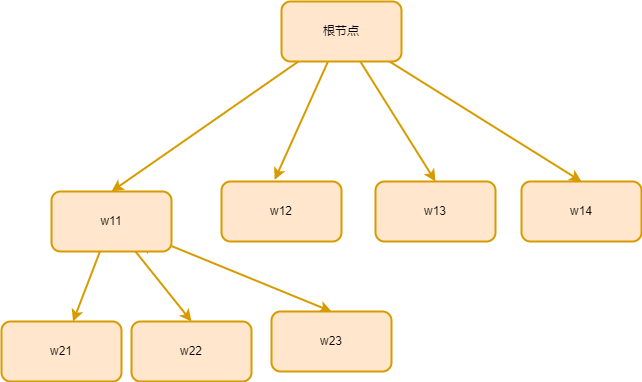# 算法第五章作业

## 1. 请用回溯法的方法分析“最小重量机器设计问题”

### 1.1 说明“最小重量机器设计问题"的解空间

(w11,w21,w31,w41)…………

(w12,w21,w31,w41)…………

…………………………………………

### 1.2 说明 “最小重量机器设计问题"的解空间树## 2. 你对回溯算法的理解

​​#include<bits/stdc++.h>using namespace std;int n,m,d;int array1;//cijint array2;//wijint cw=0;int cp=0;int bestw=1000000;int x;//记录所选部门int x1;void machine(int t){    if(t>=n){        if(cw<bestw){            bestw=cw;            for(int i=0;i<n;i++){                x1[i]=x[i];            }        }        return;    }   for(int i=0;i<m;i++){       cp+=array1[t][i];       cw+=array2[t][i];       x[t]=i;      if(cp<=d && cw <=bestw){        machine(t+1);      }      cp-=array1[t][i];      cw-=array2[t][i];   }}int main(){​•    cin >> n >> m >> d;•    memset(x1,0,sizeof(x1));•    memset(x,0,sizeof(x));•    memset(array1,0,sizeof(array1));•    memset(array2,0,sizeof(array2));•    for(int i=0;i<n;i++){•        for(int j=0;j<m;j++){•            cin >> array1[i][j];•        }•    }•     for(int i=0;i<n;i++){•        for(int j=0;j<m;j++){•            cin >> array2[i][j];•        }•    }•    machine(0);•    cout << bestw << endl;•     for(int i=0;i<n;i++){•        cout << x1[i]+1 << " ";•     }•    return 0;​}

posted @ 2021-12-14 23:30  半个一加冰  阅读(28)  评论(0编辑  收藏  举报